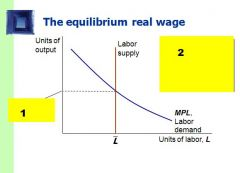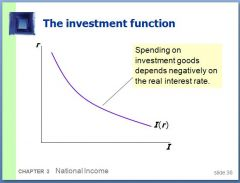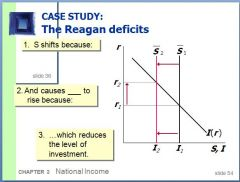• Shuffle
Toggle On
Toggle Off
• Alphabetize
Toggle On
Toggle Off
• Front First
Toggle On
Toggle Off
• Both Sides
Toggle On
Toggle Off
Toggle On
Toggle Off
Front

## Card Range To Study

throughPlay buttonPlay buttonProgress

1/46

Click to flip

Use LEFT and RIGHT arrow keys to navigate between flashcards;

Use UP and DOWN arrow keys to flip the card;

H to show hint;

 Labor (L) and capital (K) are considered the _____ of ______. Factors of Production What is the production function? Y = F(K,L) What is assumed about labor and capital and, thus, output? Labor and capital are fixed and thus, output is fixed. Constant returns to scale indicate what? That for every percentage increase in input, there is an equitable increase in output. For example, adding 10% more labor increases output 10%. ______ ________ are the amounts paid to the factors of production. Factor prices. Factor prices in an economy where the two factors of production are _____ & ______ Wages; Rent Marginal Product of Labor (MPL) is what? The extra amount of output the firm gets from one extra unit of labor if everything else remains fixed. What is the MPL equation? MPL = F(K, L+1) - F(K,L) Why do most production functions have the property of diminishing marginal product? Adding additional resources does not increase productivity. Real wage is the payment of labor measured in units of what? Output rather than dollars. In the farmer and barber example, the farmer's real wage is measured in farm goods while the barber's real wage is measured in haircuts. For any given real wage, at which point does the firm stop hiring people? At the point where MPL = real wage. After that, the MPL creates diminishing returns. When does a firm stop demanding factors of production? When the factor's marginal product is equal to its real price. The national accounting identity says that what four things make up GPD? Consumption (C), Business Investment (I), Government Purchases (G), and Net Exports (NX). The income of the household after all taxes are paid. Disposable Income Consumption Function is written as: C(Y-T) What is the marginal propensity to consume? The amount by which consumption changes when disposable income increases by one dollar. How does the investment function relate interest rate (r) and investment (I)? Investment depends on the real interest rate because the interest rate is the cost of borrowing. The investment function slopes downward: when the interest rate rises, fewer investment projects are profitable. What is the difference between the nominal interest rate and the real interest rate? The nominal interest rate is quoted rate an investor invests at. The real interest rate is the nominal interest rate adjusted for inflation. True or False: government spending and taxes are, unless otherwise stated, taken exogenously. True! Savings (S) is expressed as: Y - C - G Savings (S) is also called National Savings National Savings is made up of two parts: 1) 2) 1) Private Savings 2) Public Savings Private Savings is expressed as: Y - T - C (disposable income - consumption) Public Savings is expressed as: T - G (government revenue - government spending) When G > T, a _____ exists. Deficit For FIXED VALUES of Y, G, and T, what is also fixed? Savings (S) In the equation S = I(r), the right hand of the equation shows what? Investment is dependent on the interest rate.1. Why is the labor line vertical? 2. What is this? 3. What adjusts to equate labor supply with labor demand? 1. The labor line is vertical because we assume that the supply of labor is fixed. 2. Equilibrium Wage Rate 4. Real WageWhy does investment depend negatively on the interest rate? As the interest rate increases, fewer projects are profitable. True or False: fiscal policy affects savings, investment, and the interest rate. True! Why must investment be affected if government purchases (G) increases? Investment (I) must change because: 1. Total output (Y) is fixed by the factors of production. 2. Taxes have remained the same, so Y-T is constant. Consumption (C) is constant. If (G) increases, how does it affect the interest rate? The interest rate increases, "crowding out" investment.Answer 1. and 2. about how increasing government spending affects savings and investment. 1. (S) shifts because increasing (G) did not come with a decrease in (T). Thus, public savings has been reduced. Decreasing public savings decreases national savings, (S). 2. The interest rate (r) rises to create equilibrium.Answer 1. and 2. about how increasing government spending affects savings and investment. 1. (S) shifts because increasing (G) did not come with a decrease in (T). Thus, public savings has been reduced. Decreasing public savings decreases national savings, (S). 2. The interest rate (r) rises to create equilibrium. Would the graph for a decrease in taxes (T) be similar to the graph for an increase in government spending (G)? Yes because a decrease in (T), everything else constant, increases disposable income and reduces public saving. When public saving decreases, it decreases national savings. Which two fiscal policy maneouvers "crowd out" investment? Anything that reduces savings - increasing government purchases or decreasing taxes. What is the third fiscal policy action that affects investment, interest rates, and savings? Changes in investment demand. What are two possible causes for changes in investment demand? Technological Innovation Government encourages/discourages investment through tax laws. If savings (S) is assumed to be fixed, when investment demand changes, how is the interest rate affected? It is raised. If savings (S) is based on interest rate (r), an __________ in investment demand _________ the equilibrium interest rate (r) and _______ the equilibrium (S) and (I). raise, raise, raise or decrease, decrease, decrease When savings is postively related to interest rate, there is a direct relationship between savings, interest rate, and investment demand. What determines the amount of output an economy produces? The factors of production: labor and capital. Explain how a competitive, profit-maximizing firm decides how much of each factor of production to demand. A competitive, profit-maximizing firm decides how much of each factory of production to demand by assessing the diminishing marginal product of each factor. The firm will stop demanding that particular factor when the real price of the factor equals its marginal product. What determines consumption and investment? Consumption is determined by the consumption function C(Y-T). People consume after they have paid taxes (and sometimes before, haha). Investment is determined by the interest rate and is described by the function I(r). Explain the difference between government purchases and transfer payments. Give two examples of each. Government purchases are actually the government participating in the economy as an entity, such as purchasing aircraft from Boeing or contracting engineering services from URS. Transfer payments are a function of the government like social security and welfare. They transfer benefits to people without getting anything in return. What makes the demand for the economy's output of good equal to the supply? Interest rates. Explain what happens to consumption, investment, and the interest rate when the government increases taxes. When taxes are raised, the exact opposite of crowding out happens. 1. Consumption falls. 2. Investment increases 3. Interest rates decrease.